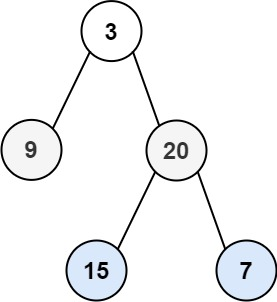0102. 二叉树的层序遍历

# 0102. 二叉树的层序遍历#

• 标签：树、广度优先搜索、二叉树
• 难度：中等

## 题目大意 #

• 返回结果为二维数组，每一层都要存为数组返回。

• 示例 1：1 2  输入：root = [3,9,20,null,null,15,7] 输出：[,[9,20],[15,7]] 
• 示例 2：
 1 2  输入：root =  输出：[ 

## 解题思路 #

### 思路 1：广度优先搜索 #

1. 判断二叉树是否为空，为空则直接返回。
2. 令根节点入队。
3. 当队列不为空时，求出当前队列长度 $s_i$。
4. 依次从队列中取出这 $s_i$ 个元素，并对这 $s_i$ 个元素依次进行访问。然后将其左右孩子节点入队，然后继续遍历下一层节点。
5. 当队列为空时，结束遍历。

### 思路 1：代码 #

  1 2 3 4 5 6 7 8 9 10 11 12 13 14 15 16 17 18 19  class Solution: def levelOrder(self, root: TreeNode) -> List[List[int]]: if not root: return [] queue = [root] order = [] while queue: level = [] size = len(queue) for _ in range(size): curr = queue.pop(0) level.append(curr.val) if curr.left: queue.append(curr.left) if curr.right: queue.append(curr.right) if level: order.append(level) return order 

### 思路 1：复杂度分析 #

• 时间复杂度：$O(n)$。其中 $n$ 是二叉树的节点数目。
• 空间复杂度：$O(n)$。